# numpy.random.noncentral_chisquare¶

numpy.random.noncentral_chisquare(df, nonc, size=None)

Draw samples from a noncentral chi-square distribution.

The noncentral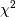distribution is a generalisation of thedistribution.

Parameters: df : int Degrees of freedom, should be >= 1. nonc : float Non-centrality, should be > 0. size : int or tuple of ints, optional Output shape. If the given shape is, e.g., (m, n, k), then m * n * k samples are drawn. Default is None, in which case a single value is returned.

Notes

The probability density function for the noncentral Chi-square distribution is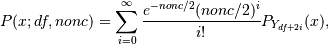where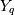is the Chi-square with q degrees of freedom.

In Delhi (2007), it is noted that the noncentral chi-square is useful in bombing and coverage problems, the probability of killing the point target given by the noncentral chi-squared distribution.

References

 [R214] Delhi, M.S. Holla, “On a noncentral chi-square distribution in the analysis of weapon systems effectiveness”, Metrika, Volume 15, Number 1 / December, 1970.
 [R215] Wikipedia, “Noncentral chi-square distribution” http://en.wikipedia.org/wiki/Noncentral_chi-square_distribution

Examples

Draw values from the distribution and plot the histogram

>>> import matplotlib.pyplot as plt
>>> values = plt.hist(np.random.noncentral_chisquare(3, 20, 100000),
...                   bins=200, normed=True)
>>> plt.show()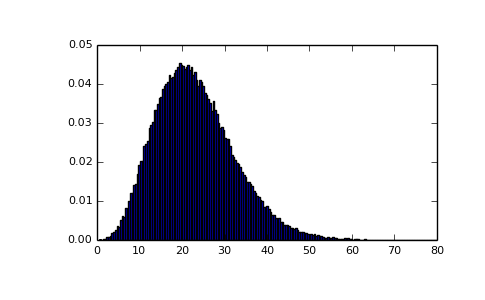Draw values from a noncentral chisquare with very small noncentrality, and compare to a chisquare.

>>> plt.figure()
>>> values = plt.hist(np.random.noncentral_chisquare(3, .0000001, 100000),
...                   bins=np.arange(0., 25, .1), normed=True)
>>> values2 = plt.hist(np.random.chisquare(3, 100000),
...                    bins=np.arange(0., 25, .1), normed=True)
>>> plt.plot(values[0:-1], values-values2, 'ob')
>>> plt.show()


(png, pdf)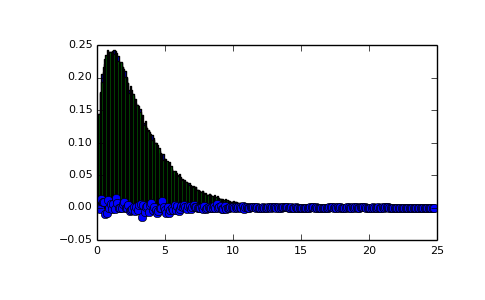Demonstrate how large values of non-centrality lead to a more symmetric distribution.

>>> plt.figure()
>>> values = plt.hist(np.random.noncentral_chisquare(3, 20, 100000),
...                   bins=200, normed=True)
>>> plt.show()


(png, pdf)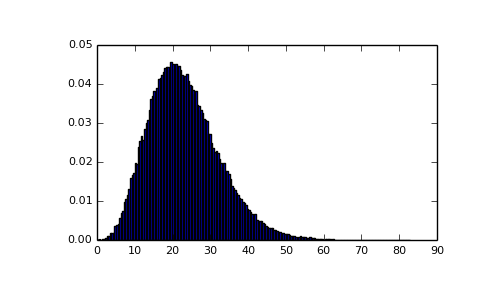#### Previous topic

numpy.random.negative_binomial

#### Next topic

numpy.random.noncentral_f